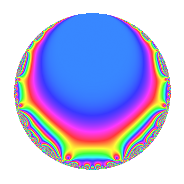# Properties

 Label 5610.2.a.bsLevel 5610 Weight 2 Character orbit 5610.a Self dual Yes Analytic conductor 44.796 Analytic rank 1 Dimension 2 CM No Inner twists 1

# Related objects

## Newspace parameters

 Level: $$N$$ = $$5610 = 2 \cdot 3 \cdot 5 \cdot 11 \cdot 17$$ Weight: $$k$$ = $$2$$ Character orbit: $$[\chi]$$ = 5610.a (trivial)

## Newform invariants

 Self dual: Yes Analytic conductor: $$44.7960755339$$ Analytic rank: $$1$$ Dimension: $$2$$ Coefficient field: $$\Q(\sqrt{13})$$ Coefficient ring: $$\Z[a_1, \ldots, a_{7}]$$ Coefficient ring index: $$2$$ Fricke sign: $$1$$ Sato-Tate group: $\mathrm{SU}(2)$

## $q$-expansion

Coefficients of the $$q$$-expansion are expressed in terms of $$\beta = \sqrt{13}$$. We also show the integral $$q$$-expansion of the trace form.

 $$f(q)$$ $$=$$ $$q - q^{2} + q^{3} + q^{4} - q^{5} - q^{6} + ( 1 + \beta ) q^{7} - q^{8} + q^{9} +O(q^{10})$$ $$q - q^{2} + q^{3} + q^{4} - q^{5} - q^{6} + ( 1 + \beta ) q^{7} - q^{8} + q^{9} + q^{10} - q^{11} + q^{12} + ( -3 - \beta ) q^{13} + ( -1 - \beta ) q^{14} - q^{15} + q^{16} + q^{17} - q^{18} + ( -3 - \beta ) q^{19} - q^{20} + ( 1 + \beta ) q^{21} + q^{22} + ( -1 + \beta ) q^{23} - q^{24} + q^{25} + ( 3 + \beta ) q^{26} + q^{27} + ( 1 + \beta ) q^{28} + 6 q^{29} + q^{30} + ( -2 - 2 \beta ) q^{31} - q^{32} - q^{33} - q^{34} + ( -1 - \beta ) q^{35} + q^{36} + 2 \beta q^{37} + ( 3 + \beta ) q^{38} + ( -3 - \beta ) q^{39} + q^{40} + ( 2 - 2 \beta ) q^{41} + ( -1 - \beta ) q^{42} + ( -9 - \beta ) q^{43} - q^{44} - q^{45} + ( 1 - \beta ) q^{46} + 12 q^{47} + q^{48} + ( 7 + 2 \beta ) q^{49} - q^{50} + q^{51} + ( -3 - \beta ) q^{52} + ( -5 - \beta ) q^{53} - q^{54} + q^{55} + ( -1 - \beta ) q^{56} + ( -3 - \beta ) q^{57} -6 q^{58} + ( -7 + \beta ) q^{59} - q^{60} + 2 q^{61} + ( 2 + 2 \beta ) q^{62} + ( 1 + \beta ) q^{63} + q^{64} + ( 3 + \beta ) q^{65} + q^{66} -10 q^{67} + q^{68} + ( -1 + \beta ) q^{69} + ( 1 + \beta ) q^{70} + ( -5 - \beta ) q^{71} - q^{72} + ( -5 + \beta ) q^{73} -2 \beta q^{74} + q^{75} + ( -3 - \beta ) q^{76} + ( -1 - \beta ) q^{77} + ( 3 + \beta ) q^{78} + ( -3 - \beta ) q^{79} - q^{80} + q^{81} + ( -2 + 2 \beta ) q^{82} + ( 1 + \beta ) q^{84} - q^{85} + ( 9 + \beta ) q^{86} + 6 q^{87} + q^{88} + 6 q^{89} + q^{90} + ( -16 - 4 \beta ) q^{91} + ( -1 + \beta ) q^{92} + ( -2 - 2 \beta ) q^{93} -12 q^{94} + ( 3 + \beta ) q^{95} - q^{96} + ( -6 + 2 \beta ) q^{97} + ( -7 - 2 \beta ) q^{98} - q^{99} +O(q^{100})$$ $$\operatorname{Tr}(f)(q)$$ $$=$$ $$2q - 2q^{2} + 2q^{3} + 2q^{4} - 2q^{5} - 2q^{6} + 2q^{7} - 2q^{8} + 2q^{9} + O(q^{10})$$ $$2q - 2q^{2} + 2q^{3} + 2q^{4} - 2q^{5} - 2q^{6} + 2q^{7} - 2q^{8} + 2q^{9} + 2q^{10} - 2q^{11} + 2q^{12} - 6q^{13} - 2q^{14} - 2q^{15} + 2q^{16} + 2q^{17} - 2q^{18} - 6q^{19} - 2q^{20} + 2q^{21} + 2q^{22} - 2q^{23} - 2q^{24} + 2q^{25} + 6q^{26} + 2q^{27} + 2q^{28} + 12q^{29} + 2q^{30} - 4q^{31} - 2q^{32} - 2q^{33} - 2q^{34} - 2q^{35} + 2q^{36} + 6q^{38} - 6q^{39} + 2q^{40} + 4q^{41} - 2q^{42} - 18q^{43} - 2q^{44} - 2q^{45} + 2q^{46} + 24q^{47} + 2q^{48} + 14q^{49} - 2q^{50} + 2q^{51} - 6q^{52} - 10q^{53} - 2q^{54} + 2q^{55} - 2q^{56} - 6q^{57} - 12q^{58} - 14q^{59} - 2q^{60} + 4q^{61} + 4q^{62} + 2q^{63} + 2q^{64} + 6q^{65} + 2q^{66} - 20q^{67} + 2q^{68} - 2q^{69} + 2q^{70} - 10q^{71} - 2q^{72} - 10q^{73} + 2q^{75} - 6q^{76} - 2q^{77} + 6q^{78} - 6q^{79} - 2q^{80} + 2q^{81} - 4q^{82} + 2q^{84} - 2q^{85} + 18q^{86} + 12q^{87} + 2q^{88} + 12q^{89} + 2q^{90} - 32q^{91} - 2q^{92} - 4q^{93} - 24q^{94} + 6q^{95} - 2q^{96} - 12q^{97} - 14q^{98} - 2q^{99} + O(q^{100})$$

## Embeddings

For each embedding $$\iota_m$$ of the coefficient field, the values $$\iota_m(a_n)$$ are shown below.

For more information on an embedded modular form you can click on its label.

Label $$\iota_m(\nu)$$ $$a_{2}$$ $$a_{3}$$ $$a_{4}$$ $$a_{5}$$ $$a_{6}$$ $$a_{7}$$ $$a_{8}$$ $$a_{9}$$ $$a_{10}$$
1.1
 −1.30278 2.30278
−1.00000 1.00000 1.00000 −1.00000 −1.00000 −2.60555 −1.00000 1.00000 1.00000
1.2 −1.00000 1.00000 1.00000 −1.00000 −1.00000 4.60555 −1.00000 1.00000 1.00000
 $$n$$: e.g. 2-40 or 990-1000 Significant digits: Format: Complex embeddings Normalized embeddings Satake parameters Satake angles

## Inner twists

This newform does not admit any (nontrivial) inner twists.

## Atkin-Lehner signs

$$p$$ Sign
$$2$$ $$1$$
$$3$$ $$-1$$
$$5$$ $$1$$
$$11$$ $$1$$
$$17$$ $$-1$$

## Hecke kernels

This newform can be constructed as the intersection of the kernels of the following linear operators acting on $$S_{2}^{\mathrm{new}}(\Gamma_0(5610))$$:

 $$T_{7}^{2} - 2 T_{7} - 12$$ $$T_{13}^{2} + 6 T_{13} - 4$$ $$T_{19}^{2} + 6 T_{19} - 4$$ $$T_{23}^{2} + 2 T_{23} - 12$$